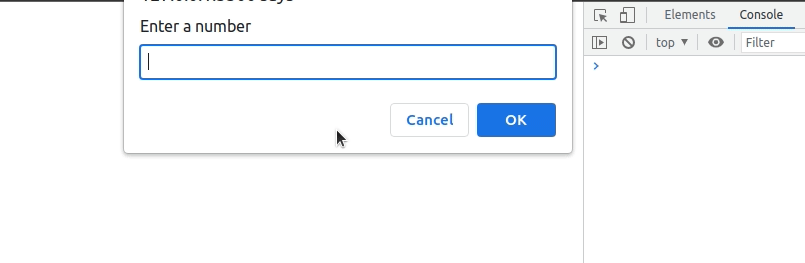Open in App
Not now

# How does Implicit coercion differs from Explicit coercion in JavaScript ?

• Last Updated : 30 Nov, 2022

Coercion refers to the process of automatic or implicit conversion of values from one data type to another. This includes conversion from Number to String, String to Number, Boolean to Number, etc. when different types of operators are applied to the values.

There are two types of coercion, Implicit coercion and explicit coercion:

1. Implicit coercion: Implicit coercion refers to JavaScript attempting to coerce an unexpected value type to the expected type. So we can pass a string where it expects a number, an object where it expects a string, etc, and it will try to convert it to the right type.

Example 1: Below is an example that will demonstrate implicit coercion:

## Javascript

 ``

Output:

```8 string
6 'number'```

Explanation: In the above code, we are initializing x and setting 8 (number). Then we are adding empty string (” “)to the number value which will implicitly convert the number into a string as the ‘+’ operator implicitly converts the number into a string. So, the final output will be of string type.

Then in the next line, we are subtracting 2 from the string x (the number is converted into a string). The ‘-‘ operator will implicitly convert the string into a number and then perform the operation. So, 2 will be subtracted from 8 and the final output will be 6 of the number type.

Example 2: This is another example of implicit coercion:

## Javascript

 ``

Output:

`Number`

Explanation: In this case, the data type of the variable is automatically converted to a number. That’s what implicit conversion is.

2. Explicit coercion: Explicit coercion refers to changing the data type of the variable by the user itself depending upon the type in which we want to convert it.

Example 1: Below is an example that will demonstrate explicit coercion:

## Javascript

 ``

Output:

`0`

Explanation: In our example, we used the Number() function with an empty string and received a 0.

Example 2: In the below example, we are using a prompt() to demonstrate explicit coercion:

## Javascript

 ``

Output:Explanation: In the above code, we are using prompt() and taking value from the user. Now by default, the prompt() takes a value in string type. So we need to explicitly convert that string into a number according to the requirement.

Difference between Implicit and Explicit coercion:

My Personal Notes arrow_drop_up
Related Articles